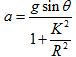×#### Thank you for registering.

One of our academic counsellors will contact you within 1 working day.

Click to Chat

1800-1023-196

+91-120-4616500

CART 0

• 0

MY CART (5)

Use Coupon: CART20 and get 20% off on all online Study Material

ITEM
DETAILS
MRP
DISCOUNT
FINAL PRICE
Total Price: Rs.

There are no items in this cart.
Continue Shopping
```
Three rolling objects are moving at the same speed on a level surface. The objects are all solid spheres: sphere A has radius r and mass m, sphere B has radius 2r and mass m; sphere C has radius r and mass 2m.The three objects then roll up an incline. Assuming each rolls without slipping, which will (a) roll to the highest vertical point (as measured by the change in the location of the center of mass)? (A) Sphere A (B) Sphere B (C) Sphere C (D) They will all roll to the same height. (b) roll the farthest distance as measured along the incline? (A) Sphere A (B) Sphere B (C) Sphere C (D) They will all roll the same distance.
Three rolling objects are moving at the same speed on a level surface. The objects are all solid spheres: sphere A has radius r and mass m, sphere B has radius 2r and mass m; sphere C has radius r and mass 2m.The three objects then roll up an incline. Assuming each rolls without slipping, which will(a) roll to the highest vertical point (as measured by the change in the location of the center of mass)?(A) Sphere A (B) Sphere B (C) Sphere C (D) They will all roll to the same height.(b) roll the farthest distance as measured along the incline? (A) Sphere A (B) Sphere B (C) Sphere C(D) They will all roll the same distance.

```
5 years ago

```							(a) The correct option is (B) sphere B.Acceleration a of a body rolling down an inclined plane will be,Here g is the free fall acceleration, K is the radius of gyration, θ is the angle of inclination with horizontal and R is the radius of the body.The above equation signifies that, the object which has radius more, the more will be the acceleration of that object and the acceleration of the object does not depend upon the mass of the object M. As the radius of sphere B is more than the sphere A and C, therefore the acceleration of sphere B will be more than A and C. Thus sphere B roll to the highest vertical point. From the above observation we conclude that, option (B) is correct.(b)The correct option is (B) sphere B.The object which has greater acceleration greater will be roll to the highest vertical point. Thus this object will roll the farthest distance as measured along the incline. As the sphere B roll to the highest vertical point, therefore sphere B will roll to the farthest distance as measured along the incline. From the above observation we conclude that, option (B) is correct.
```
5 years ago
Think You Can Provide A Better Answer ?

## Other Related Questions on Mechanics

View all Questions »### Course Features

• 101 Video Lectures
• Revision Notes
• Previous Year Papers
• Mind Map
• Study Planner
• NCERT Solutions
• Discussion Forum
• Test paper with Video Solution### Course Features

• 110 Video Lectures
• Revision Notes
• Test paper with Video Solution
• Mind Map
• Study Planner
• NCERT Solutions
• Discussion Forum
• Previous Year Exam Questions